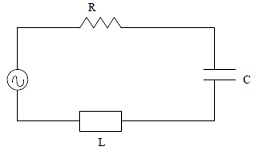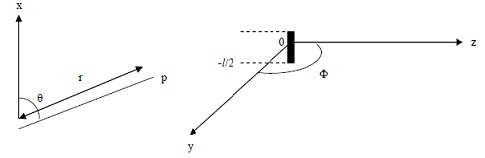#### Resonant Circuit, Physics tutorial

Resonant circuit:

Resonance is a phenomenon in which a vibrating system responds with maximum amplitude to an alternating driving (external) force. Thus any electrical circuit that exhibit resonance is a resonant circuit.Resonance occurs when the frequency of the external or driving force is exactly equal to the natural frequency of the undamped system. The natural frequency of the undamped system is determined from the circuit above in the absence of the resistance which causes damping. The equation of motion for such a free oscillatory circuit when no external force is applied is

L(di/dt) + q/c = 0 or L(d2q/dt2) + q/c = 0

Solution of this differential equation is

q = q0sinw0t

Where q is charge at any point in time, t and qo is maximum value of charge while w, is angular frequency of oscillation. By differentiating equation twice, we get

d2q/dt2 = -w2q0sinwt

This equal to d2q/dt2 = -w2q or d2/dt2 + w2q = 0

Comparing equation

w2 = 1/LC or w = 1/√LC

Implying that natural angular frequency, w, equals 1/√LC

Impedance, Z, of RLC circuit

Z = √[R2 + (XL + Xc)2]

Where XL, inductive reactance (positive reactance) = wL and XC, capacitance reactance (negative reactance) = 1/wc. Substituting equation becomes Z = √R2 + [((wL) - (1/wc)]2

When wL = 1/wc

Current flowing will be high from Irms = Erms/Z and resonance occurs. Circuit is then said to be tuned to resonant frequency. From equation

w = 1/√LC

When resonance takes place (compare equations (4) and (8)). Tuning of radio set is demonstration of resonance in which knob of receiver-set is utilized to differ either inductance or capacitance such that natural frequency of the set is equal to frequency of desired signal. In this way different radio signals of choice can be received.

A dipole is system of two equal but opposite charges separated by short distance. Product of distance, x and magnitude of one of the charges, q is dipole moment, P. We can then write

P = qx

Radio waves are produced by oscillating electrons in conductors and dipole antennae. Example of such a dipole is that of two brass rods with small gap between is joined to high voltage supply, say 5kv. Oscillatory discharge between polished ends of rods is generated. This sparkling is because of ionization of air in gap between ends of rods because of magnitude of electric field. Two brass rods explain antenna or aerial. Antennas are frequently in form of metal wire or rod in which electrons surge backward and forward periodically. At a certain time, one end of wire or rod will be negative and other end positive. Half a cycle later polarity of ends is exactly reversed. This is referred to as the oscillating electric dipole as its dipole moment changes in periodic way with time.

Oscillating dipole also radiates electromagnetic field in radio wave frequency range. Radiation field of the oscillating electric dipole is attained as follows. Let charge of spark differ with time as q = qAsinwt where qo is amplitude of oscillating charge and w is angular frequency of oscillations. Suppose gap between brass rods be small compared to wavelength of radiation generated. Current set up can be written as I = wqAcoswt = IAcoswt

Therefore I A = wqA

The oscillating charge is equal to oscillating dipole moment. Suppose gap between rods be of magnitude x in length, then dipole moment, P is P = qx = PAsinwt

Where PA = qAx

Simple oscillating dipole is known as a Hertzian dipole. Power density is given by Poynting vector. Poynting vector, N = E x B. We can also write P = E x B. We need to determine expressions of electric (E) and magnetic fields (B) of radiation. Consider very simple antenna i.e. short filament in which current I = Iosinwt is flowing. Magnetic field is induced in neighborhood of filament. The radiation is electromagnetic.

Components of electromagnetic field at a point P, a distance r, from centre are to be determined.The field at P will be generated by current flowing through filament at time t - r/c, where r/c is time taken for field to travel from filament to P at velocity of light.

Retarded potential A, is related to current in filament by A = 1/4π∫v i[(t - (r/c))]/r]dv

As current is in x-axis only we can write Ax = 1/4π 1/21/2[(Isinw[t - (r/c))/r]dx = (Il/4πr)sinw[t-(r/c)]. It can be illustrated that retarded potential A is related to magnetic field B, by B = ∇xA

Using Spherical coordinate, Ar = Axcosθ = (Il/4πr)sinw(t -(r/c))cosθ

Aθ = -Axsinθ = -(Il/4πr)sinw(t-(r/c))sinθ, AΦ = 0

Using equation we can find spherical components of magnetic fields: Br = 0, Bθ = 0

BΦ = (Il/4π)sin[(w/cr)cosw(t-r/c) + (1/r2)sinw(t-r/c)]

Spherical components of electric fields are obtained from differential form of Faraday's law i.e. third Maxwell's equation i.e ∇xE = -dB/dt, Er = 0

Eθ = -[wIlsinθ/4πε0c2](sin[w(t-r/c)]/r), EΦ = 0

Moving charges are known as electric currents. Thus a wire in which charges flow is an electric current-carrying-wire. Around such wires or conductors, radiation is generated. In the last section, the radiation from oscillating dipole was treated. This radiation consists of radio waves. When electrons are made to oscillate at frequencies of 10GHz in a tuned cavity, microwaves (with wavelength of a few centimetres) are produced. The devices used to cause the oscillations of charge which produce microwaves are magnetrons or klystrons. These devices use resonant cavities. Simply defined, resonant cavity is a piece of waveguide closed off at both ends. Whereas wave is propagated in a longitudinal direction in the waveguide, in the resonant cavity standing waves exist and oscillations can take place if the cavity resonator - as it is also called - is suitably excited. The simplest cavity whose resonant frequencies can be calculated is a rectangular cavity.

In the rectangular cavity resonator, the electric field can be represented by E = E0f(x,y,z)exp(jwt)

Where w is angular frequency of wave function f(x,y,z) gives variation of electric field in three directions. Note that electric field is no longer constant in z-direction i.e. it now differs in that direction.

Following example of waveguide components of electric field of TE standing waves in cavity that satisfy boundary conditions on walls and satisfy equation ∇.E = 0 are

Ex = -(cnπ/b)cos(mπx/a)sin(nπy/b)sin(lπz/d)exp(jwt)

Ex = (cmπ/a)sin(mπx/a)cos(nπy/b)sin(lπz/d)exp(jwt)

Ez = 0

Associated magnetic field components are obtainable from differential form of Faraday's law i.e. ∇.E = -dB/dt or by using pattern of magnetic field component of rectangular waveguide. Magnetic field components are

By = (-jknlπ2/wbd)sin(nπy/b)cos(mπx/a)cos(lπz/d)exp(jwt)

Bz = jkπ2/w[(m/a)2 + (n/b)2]cos(mπx/a)cos(nπy/b)sin(lπz/d)exp(jwt)

These electric field and magnetic field components would be acceptable solutions to Maxwell's equations inside cavity if they satisfy wave equations for electric and magnetic fields. Substituting electric field and magnetic components into suitable wave equations shows that fields are acceptable. If the integers m, n, l and wave number k are related by

π2m2/a2 + π2n2/b2 + π2l2/d2 = k2

Which resonant frequencies (depending on integers m, n, l) of cavity can be derived as follows. We can write

22 = π2m2/a2 + π2n2/b2 + π2l2/d2 and using λ = c/f, we have

4f2/c2 = m2/a2 + n2/b2 + l2/d2 or f = c/2√[(m/a)2 + (n/b)2 + (l/d)2]

Lowest frequency - in which lowest mode is excited - corresponds to any two of m, n and l equals to one and third integer associated with shortest side of cavity, equal to zero i.e. TE011, TE101, or TE110 as case may be. Another set of possible solutions for electromagnetic fields in cavity are expressions of electric and magnetic fields corresponding to Transverse Magnetic (TM) standing waves in which electric field has z-component while z-component of magnetic field is zero. TMmnl waves take place at same frequencies as TEmnl waves for same cavity resonator. Sum of resonating TE and TM waves provides particular resonance of standing wave.

Cavity resonator can be utilized as frequency meters apart from its use for generation of microwaves. Measurement of frequency is made possible by use of plunger attached to one end face which then permits resonant length of cavity to differ.

Tutorsglobe: A way to secure high grade in your curriculum (Online Tutoring)